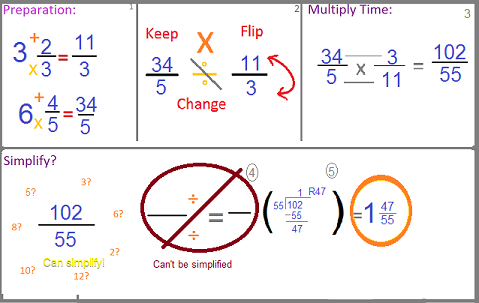# Dividing Compound Fractions

Instructor: Christine Quist

Christine has taught 4th-5th grade, has worked as a Paraprofessional for Adult Learners, and has a master's degree in Curriculum and Instruction for Elementary Education.

In this lesson, we will learn the steps necessary to divide compound fractions. Dividing fractions is only one step away from multiplying, so it's easy!

## Compound Fractions

A compound fraction has a whole number and a fraction. A whole number is like one whole cookie, unbroken, and all for you! When you get a fraction like 1/2 (one half) or 1/3 (one third), then you start breaking that whole cookie into pieces and sharing them with your friends. If you split your cookie into three parts and you only get one of them, then you got 1/3 of the whole cookie. Dividing fractions is very close to multiplying fractions: it's just a KEEP, CHANGE, FLIP!

## From Compound to Improper

Let's say you have to divide 6 4/5 by 3 2/3. To begin, you will need to change the compound fractions, also known as mixed numbers, into improper fractions, which are when the number on top is larger than the number on the bottom. This happens when you add a whole number into the fraction. To convert a compound fraction into an improper fraction, you will need to use these two steps:

Step 1: Take 3 times the bottom number, in this case the 3. 3 x 3 = 9

Step 2: Take the 9 and add it to the top number 9 + 2 = 11

Answer: This number replaces the top number and creates the improper fraction of 11/3

The same will happen to the other fraction in the problem 6 4/5:

Step 1: The 6 will be multiplied by the bottom number of 5 = 30

Step 2: Add 30 to the top number of 4 = 34

Answer: The improper fraction is 34/5

The next step is dividing the fractions!

## Keep, Change, Flip

Lay out your new problem of 34/5 divided by 11/3:

KEEP -- To start, you will keep the first fraction as it is, do not change anything about it!

CHANGE -- Change the division sign to multiplication.

FLIP -- Flip the 11/3 so it is now 3/11

After this, you will multiply across the top numbers in the fraction and then across the bottom like in the picture.

34/5 * 3/11 = (34 x 3 = 102) and (5 x 11 = 55) which means the new fraction is 102/55.Next Step: Simplify! We need to decide if there is a number that can divide into both numbers to make the fraction smaller. 55 and 102 can both be divided by numbers on their own, but the same number cannot go into both of them. Therefore, this fraction is simplified as far as it can go.

To unlock this lesson you must be a Study.com Member.

### Register to view this lesson

Are you a student or a teacher?

#### See for yourself why 30 million people use Study.com

##### Become a Study.com member and start learning now.
Back
What teachers are saying about Study.com

### Earning College Credit

Did you know… We have over 200 college courses that prepare you to earn credit by exam that is accepted by over 1,500 colleges and universities. You can test out of the first two years of college and save thousands off your degree. Anyone can earn credit-by-exam regardless of age or education level.